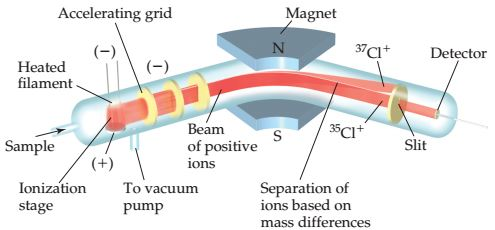# Problem: Consider the mass spectrometer show below.Determine whether each of the following statements is true or false.For a given element, the number of peaks in the spectrum is equal to the number of naturally occurring isotopes of that element.

###### FREE Expert Solution

We are asked to determine whether the following statement is true or false:

For a given element, the number of peaks in the spectrum is equal to the number of naturally occurring isotopes of that element.

94% (257 ratings)###### Problem Details

Consider the mass spectrometer show below.Determine whether each of the following statements is true or false.

For a given element, the number of peaks in the spectrum is equal to the number of naturally occurring isotopes of that element.